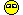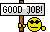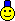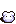Replies: 92 (Who?), Viewed: 214862 times.
Page 3 of 4Field Researcher#5127th Mar 2007 at 2:10 AM
Quote:
 Originally Posted by Citysim Just click on the Image data first then you get the sim portrait to verify the right one, eventually you will remember the characters numbers as you gotta go back soon after they age transist anyway.

FYI If you go into SimPE and into sim surgury instead, I beleave it gives you the character # for each of your sims, so you only have to load SimPE once, write down all the character # and name of the sims you want to change.
That would be even easyer.
I know this thread was started a while ago and may be a waist of my time posting this, or maby someone has already said something, but once I have read everything I will have forgotton to post.
Hope this helps.

PS Love the idea, I have my work cut out for me now hehe.
Test Subject
#523rd Apr 2007 at 8:43 PM
Question...what do you open the characters with??? If I double-click it, and then there's an error. So what am I supposed to do???#5315th Apr 2007 at 9:10 PM
Uhhhhhh, sorry mate, I just don't get it! (i'm not exactly what you'd call smart!)

SONIC AND TOKYO MEW MEW ARE THE BEST ANIMES EVAH!
Lab Assistant
#546th Jul 2007 at 10:17 PM
Updated the height list for inches only but with with in standard height conventions; ie. 63 inches is knows as 5 foot 3 inches and is not normally refered to as 5 1/4 feet. Also removed metric. I guess someone brought up that children had default size of 4' so I showed that as part of the defaults.

hope this helps....

In = Feet + Inches = Stretch Value
--- ---------------- ----------
36 = 3 feet 0 inches = 0.5
37 = 3 feet 1 inches = 0.51
38 = 3 feet 2 inches = 0.53
39 = 3 feet 3 inches = 0.54
40 = 3 feet 4 inches = 0.56
41 = 3 feet 5 inches = 0.57
42 = 3 feet 6 inches = 0.58
43 = 3 feet 7 inches = 0.6
44 = 3 feet 8 inches = 0.61
45 = 3 feet 9 inches = 0.63
46 = 3 feet 10 inches = 0.64
47 = 3 feet 11 inches = 0.65
48 = 4 feet 0 inches = 0.67 ** this is default size for children
49 = 4 feet 1 inches = 0.68
50 = 4 feet 2 inches = 0.69
51 = 4 feet 3 inches = 0.71
52 = 4 feet 4 inches = 0.72
53 = 4 feet 5 inches = 0.74
54 = 4 feet 6 inches = 0.75
55 = 4 feet 7 inches = 0.76
56 = 4 feet 8 inches = 0.78
57 = 4 feet 9 inches = 0.79
58 = 4 feet 10 inches = 0.81
59 = 4 feet 11 inches = 0.82
60 = 5 feet 0 inches = 0.83
61 = 5 feet 1 inches = 0.85
62 = 5 feet 2 inches = 0.86
63 = 5 feet 3 inches = 0.88
64 = 5 feet 4 inches = 0.89
65 = 5 feet 5 inches = 0.9
66 = 5 feet 6 inches = 0.92 ** this is default size for teens
67 = 5 feet 7 inches = 0.93
68 = 5 feet 8 inches = 0.94
69 = 5 feet 9 inches = 0.96
70 = 5 feet 10 inches = 0.97
71 = 5 feet 11 inches = 0.99
72 = 6 feet 0 inches = 1** this is default size for adults
73 = 6 feet 1 inches = 1.01
74 = 6 feet 2 inches = 1.03
75 = 6 feet 3 inches = 1.04
76 = 6 feet 4 inches = 1.06
77 = 6 feet 5 inches = 1.07
78 = 6 feet 6 inches = 1.08
79 = 6 feet 7 inches = 1.1
80 = 6 feet 8 inches = 1.11
81 = 6 feet 9 inches = 1.13
82 = 6 feet 10 inches = 1.14
83 = 6 feet 11 inches = 1.15
84 = 7 feet 0 inches = 1.17
85 = 7 feet 1 inches = 1.18
86 = 7 feet 2 inches = 1.19
87 = 7 feet 3 inches = 1.21
88 = 7 feet 4 inches = 1.22
89 = 7 feet 5 inches = 1.24
90 = 7 feet 6 inches = 1.25
91 = 7 feet 7 inches = 1.26
92 = 7 feet 8 inches = 1.28
93 = 7 feet 9 inches = 1.29
94 = 7 feet 10 inches = 1.31
95 = 7 feet 11 inches = 1.32
96 = 8 feet 0 inches = 1.33

Not many midgets less than 3 ft....heightininches.txt (1.2 KB, 161 downloads)
Lab Assistant
#556th Jul 2007 at 10:28 PM
Here is the partially illustrated SimPE height change guide

Took me awhile to find but it was where i first learned how to do it.
Test Subject
DELETED POST
27th Jul 2007 at 2:35 AM
This message has been deleted by kikosimxl. Reason: I was being stupid
Test Subject
#5617th Sep 2007 at 2:17 AM
if i make my sims tall they look like stick ppl and are weird can this be fixed
Test Subject
#5725th Nov 2007 at 5:02 PM
Does anyone know how to change the Default height of the sims?
Test Subject
#5819th Dec 2007 at 5:55 PM
What the fuck is going on...I'm about to freaking scream. Out of no where while I continued to find my sim, when I tried to click on the age data thing, it wouldnt work. I kept trying to click. And then I got frustrated and exited out of the resource tree. And I cant get it back. Its like no matter how many times I re Installed it, it the program acts as if I saved it. Im going crazy...please help!!
#5931st Dec 2007 at 2:26 PM
Hooray, thanks for this! Now I can finally make myself in-game, a short 5'5 woman :PLab Assistant#6017th Jun 2008 at 5:35 PM Last edited by gundavolt : 23rd Jun 2008 at 10:56 AM. Reason: Figured it out!
I wanted to change some sims into dwarves, but the game won't accept the change; 0.x is turned into x ..

I suppose it's in the BV or FT expansion pack this is changed, as it was possible before, but I don't know. My dwarves looked very strange, thin and long-stretched (I laughed hard when first discovered them after changing the height)

06/23
Figured it out!
At first I wrote 0.8, which the system changed to 8.
The I tried writing 0,8 instead, which worked fine!Not sure it works for all, just the European versions or what, but it worked for meInstructor#6122nd Jun 2008 at 9:45 PM
I tried to do it but when I went to my Sim, it was in the floor and the game froze. What happened? T_T
Test Subject
#622nd Aug 2008 at 12:33 AM
Same.. I changed a Sim's height and now it freezes and crashes whenever I select that family.Lab Assistant#635th Aug 2008 at 5:25 AM
My Sims no longer even have "Age Data" as an option under the Resource Tree. Does that mean it isn't possible to do this anymore?

Sims 3Lab Assistant#6419th Aug 2008 at 4:22 AM
Ugh, I was going to try this for my Sim story, but now that quite a few people have been having problems with it, I may have to back off from the idea.
Test Subject
#653rd Sep 2008 at 6:37 PMeverything works fine for me ...
i just found out how to do this ... i went and downloaded SimPE and have gone in and out of the program and game without a problem.

i did have a problem though ... the first time i entered the new heights and went to the game everything was all messed up ... then i realized that i had adjusted the toddlers and children's heights with the adult numbers ... haha

so that led me to make the toddler conversions ... i used the average height as 2'6". i also went as low as 1 foot ... but i haven't tested that, lowest i've tested is 2'0" (its really cute!!!! more like a real 1 year old). i also went up to 3'0" and that worked fine as well ... how nice it is to have two toddlers at different sizes!!

1'0" = 0.4
1'1" = 0.43
1'2" = 0.47
1'3" = 0.5
1'4" = 0.53
1'5" = 0.57
1'6" = 0.6
1'7" = 0.63
1'8" = 0.67
1'9" = 0.7
1'10" = 0.73
1'11" = 0.77
2'0" = 0.8
2'1" = 0.83
2'2" = 0.87
2'3" = 0.9
2'4" = 0.93
2'5" = 0.97
2'6" = 1
2'7" = 1.03
2'8" = 1.07
2'9" = 1.1
2'10" = 1.13
2'11" = 1.17
3'0" = 1.2
3'1" = 1.23
3'2" = 1.27
3'3" = 1.3
3'4" = 1.33
3'5" = 1.37
3'6" = 1.4
3'7" = 1.43
3'8" = 1.47
3'9" = 1.5
3'10" = 1.53
3'11" = 1.57
4'0" = 1.6

i made child and teen conversions too ... based on the average height listed above (4'0" for the child and 5'6" for the teen). they all worked fine tooInventor#664th Sep 2008 at 5:28 PM
...and I made a Excel file to calculate the sizes...
#6720th Sep 2008 at 11:07 AM
Thanks!I truly appreciate this post. I recently began my hand at Sims movie making & was trying to figure out how to permanently keep the height differences throughout gameplay. This worked perfectly with no problems.

Thank you so much!
Test Subject
#682nd Jan 2009 at 3:52 AM
Wow, that was very easy to do. Thanks!
Test Subject
#693rd Jan 2009 at 10:56 AM
The onlyroblem I'm still having is when changing the height and I choose 1.1 forexample it changes to 11. Always. The dot wont work and then in the game my sim is extremely tall obviously. Anyone know why?
Test Subject
#707th Feb 2009 at 3:57 PM Last edited by sendkoi22 : 7th Feb 2009 at 4:52 PM.
Quote:
 Originally Posted by Nudel The onlyroblem I'm still having is when changing the height and I choose 1.1 forexample it changes to 11. Always. The dot wont work and then in the game my sim is extremely tall obviously. Anyone know why?

Maybe you should use comma instead of point. IcemanTo pointed it out on the first page of this thread.Test Subject
#717th Feb 2009 at 4:39 PM Last edited by sendkoi22 : 7th Feb 2009 at 4:41 PM. Reason: Misspelled
In case you guys don't know, if you just click 'Sim Browser' you can get ALL the character numbers you need.
Test Subject
#7229th Mar 2009 at 6:04 AM
Quote:
 Originally Posted by sendkoi22 Maybe you should use comma instead of point. IcemanTo pointed it out on the first page of this thread.Yes! I have only base Game + Nightlife and only commas works fine =D! You just have to look the left pannel. When you write "1.243", for example, you see "1243" in the left pannel, but if you put "1,243" it'll be just like this in L.P. =D!

Greaaaaaaaaaaaat tutorial!!!Lab Assistant
DELETED POST
5th Apr 2009 at 12:56 PM
This message has been deleted by Echo. Reason: Not relevant
Test Subject
#737th Apr 2009 at 4:36 AMMac
I have a mac, and I can't seem to open the characters packages. Is there a particular program suggested to open them (for Mac-users)?
Test Subject
#7425th Jun 2009 at 5:31 AM
Tried the height change and then started game and my neighborhood gone missing. Looks like I have to install the game again. Btw, i backed up my neighborhood folder but replacing back didnt do squat. I have base and nightlife.
Test Subject
#7525th Jun 2009 at 5:50 AM
Nevermind, i got it back by moving the "SIMS 2" folder out of EA games folder to the desktop, and when restarting game, the "Game" gave me back all the default neighborhood. At least I save my custom lots, now I can replace it back.

I don't really know why this height change doesn't work for me. Game engine is really picky.
 Page 3 of 4